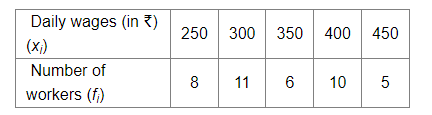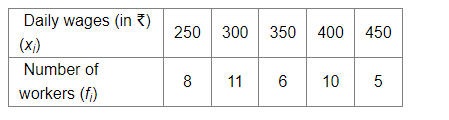# Find the mean of daily wages of 40 workers in a factory as per data given below:Question:

Find the mean of daily wages of 40 workers in a factory as per data given below:Solution:

We know that,

Mean $=\frac{\sum x_{i} f_{i}}{\sum f_{i}}$

For the following data:Mean $=\frac{(250 \times 8)+(300 \times 11)+(350 \times 6)+(400 \times 10)+(450 \times 5)}{8+11+6+10+5}$

$=\frac{2000+3300+2100+4000+2250}{40}$

$=\frac{13650}{40}$

$=341.25$

Hence, the mean of daily wages of 40 workers in a factory is 341.25 .